Courses

# NCERT Exemplar - Waves (Part- 1) Notes | EduRev

## JEE : NCERT Exemplar - Waves (Part- 1) Notes | EduRev

The document NCERT Exemplar - Waves (Part- 1) Notes | EduRev is a part of the JEE Course Physics Class 11.
All you need of JEE at this link: JEE

MULTIPLE CHOICE QUESTIONS I

Q.1. Water waves produced by a motor boat sailing in water are
(a) Neither longitudinal nor transverse.
(b) Both longitudinal and transverse.
(c) Only longitudinal.
(d) Only transverse.
Ans
. (b)
Solution.
Water waves produced by a motorboat sailing on the surface of deep water are both longitudinal and transverse because the waves, produce transverse as well as lateral vibrations in the particles of the medium. The water molecules at the surface move up and down; and back and forth simultaneously describing nearly circular paths as shown in Figure.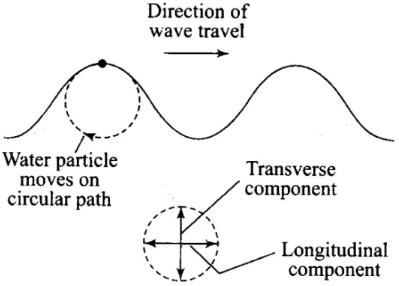As the wave passes, water molecules at the crests move in the direction of the wave while those at the troughs move in the opposite direction.

Q.2. Sound waves of wavelength λ travelling in a medium with a speed of v m/s enter into another medium where its speed is 2v m/s. Wavelength of sound waves in the second medium is
(a) λ
(b) λ/2
(c) 2λ
(d) λ
Ans.
(c)
Solution.
Frequency remains unchanged in both the medium.
So,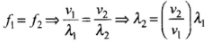λ1 and λ2 are wavelengths and v1 and v2 are speeds in first and second medium respectively.
So,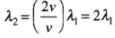Hence the wavelength of sound waves in the second medium is 2λ.

Q.3. Speed of sound wave in air
(a) Is independent of temperature.
(b) Increases with pressure.
(c) Increases with increase in humidity.
(d) Decreases with increase in humidity.
Ans.
(c)
Solution.
We know that speed of sound in air is given by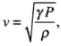for air γ ans P are constants.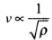where ρ is the density of air.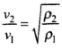where ρ1 is density of dry air and ρis density of moist air. Due to the presence of moisture, density of air decreases.
As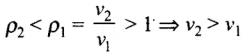Hence, speed of sound wave in air increases with increase in humidity.

Q.4. Change in temperature of the medium changes
(a) Frequency of sound waves.
(b) Amplitude of sound waves.
(c) Wavelength of sound waves.
(d) Loudness of sound waves.
Ans.
(c)
Solution.
Speed of sound wave in medium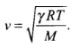Here γ, R and M are constant.
Hence, v ∝ √T
(where T is temperature of the medium)
It means when temperature changes, speed also changes.
As, v = fλ, where f is frequency and λ is wavelength.
As frequency (f) remains fixed, v ∝ λ or v ∝ v
Hence wavelength (λ) changes.

Q.5. With propagation of longitudinal waves through a medium, the quantity transmitted is
(a) Matter
(b) Energy
(c) Energy and matter
(d) Energy, matter and momentum
Ans.
(b)
Solution.
A wave is a disturbance which propagates energy and momentum from one place to the other without the transport of matter. In propagation of longitudinal waves through a medium leads to transmission of energy through the medium without matter being transmitted. There is no movement of matter (mass) and hence momentum.
Important point:
Characteristics of wave motion:

• It is a sort of disturbance which travels through a medium. ,
• Material medium is essential for the propagation of mechanical waves.
• When a wave motion passes through a medium, particles of the medium only vibrate simple harmonically about their mean position. They do leave their position and move with the disturbance.
• There is a continuous phase difference amongst successive particles of the medium, i.e. particle 2 starts vibrating slightly later than particle 1 and so on.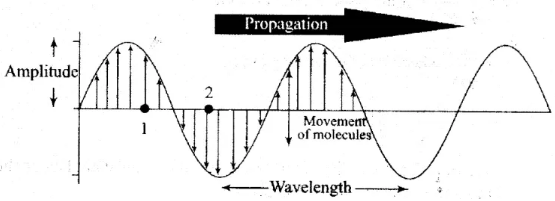• The velocity of the particle during there vibration is different at different positions different positions.
• The velocity of wave motion through a particular medium is constant. It depends only on die nature of medium not op the frequency, wavelength or intensity,
• Energy is, propagated along with the wave motion without any net transport of the medium.

Q.6. Which of the following statements are true for wave motion?
(a) Mechanical transverse waves can propagate through all mediums.
(b) Longitudinal waves can propagate through solids only.
(c) Mechanical transverse waves can propagate through solids only.
(d) Longitudinal waves can propagate through vacuum.
Ans.
(c)
Solution.
In case of mechanical transverse wave propagates through a medium, the medium particles oscillate right angles to the direction of wave motion or energy propagation. It travels in the form of crests and troughs.
When mechanical transverse wave propagates through a medium element of the medium is subjected to shearing stress. Solids and strings have shear modulus, that is why, sustain shearing stress. Fluids have no shape of their own, they yield to shearing stress. Transverse waves can be transmitted through solids, they can be setup on the surface of liquids. But they cannot be transmitted into liquids and gases.

Q.7. A sound wave is passing through air column in the form of compression and rarefaction. In consecutive compressions and rarefactions,
(a) Density remains constant.
(b) Boyle’s law is obeyed.
(c) Bulk modulus of air oscillates.
(d) There is no transfer of heat.
Ans.
(d)
Solution.

• When sound wave travels through a medium, say air, the particles of medium disturb in the same fashion, i.e. compression and rarefaction (depression). When air particles come closer it is called compression. On the other hand, when particles go farther than their normal position it is called rarefaction. In the condition of compression, molecules of medium come closer to each other and in the condition of rarefaction, molecules of the medium go farther from each other; compared to their normal positions.
• When compression takes place in the medium, the density and pressure of the medium increase. When rarefaction takes place in the medium, density and pressure of the medium decrease. This increase and decrease in density and pressure are temporary. Thus, compression is called the region of high density and pressure. Rarefaction is called the region of low density and pressure.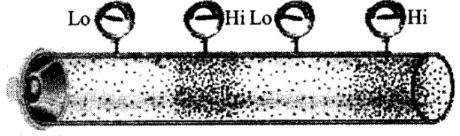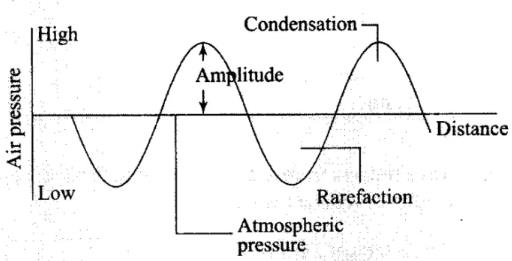Q.8. Equation of a plane progressive wave is given by y = 0.6 sin 2π(t - x/2). On reflection from a denser medium its amplitude becomes 2/3 of the amplitude of the incident wave. The equation of the reflected wave is
(a)  y = 0.6 sin 2π(t + x/2)
(b)  y = -0.4 sin 2π(t + x/2)
(c)  y = 0.4 sin 2π(t + x/2)
(d)  y = -0.4 sin 2π(t - x/2)
Ans.
(b)
Solution.
Reflection of Mechanical Waves: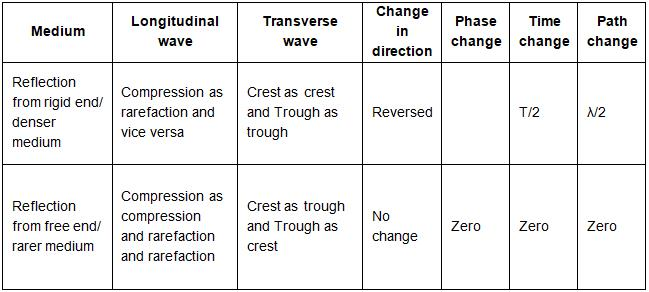When a wave reflects from a denser medium, there is a phase change of π.
Given equation of incident wave
yi = 0.6 sin 2π(t - x/2)
Equation of reflected wave is
yr = Ar sin 2π(t + x/2 + π)
Amplitude of reflected wave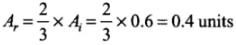So,   yr = - 0.4 sin 2π (t + x/2 )

Q.9. A string of mass 2.5 kg is under a tension of 200 N. The length of the stretched string is 20.0 m. If the transverse jerk is struck at one end of the string, the disturbance will reach the other end in
(a) 1 second
(b) 0.5 second
(c) 2 seconds
(d) Data given is insufficient
Ans.
(b)
Solution.
The speed of transverse wave in any string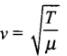μ = mass per unit length
Mass m = 2.5 kg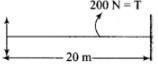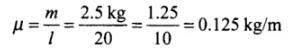Hence wave speed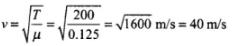Time taken by the disturbance to travel from one end to the other end of the string i.e., to travel a distance of 20m is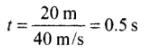Q.10. A train whistling at constant frequency is moving towards a station at a constant speed V. The train goes past a stationary observer on the station. The frequency n of the sound as heard by the observer is plotted as a function of time t (Figure) . Identify the expected curve.
(a)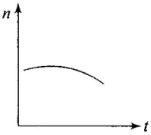(b)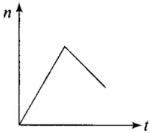(c)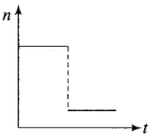(d)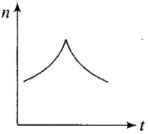Ans.
(c)
Solution.
General expression for Apparent Frequency. Suppose observer (O) and source (S) are moving in the same direction along a line with velocities v0 and vs respectively. Velocity of sound is v and velocity of medium is vm, then apparent frequency observed by observer is given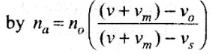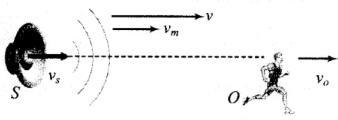If medium is stationary, i.e., vm = 0, then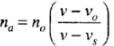Sign convention for different situations:

• The direction of v is always taken from source to observer.
• All the velocities in the direction of v are taken positive.
• All the velocities in the opposite direction of v are taken negative.

When train is approaching towards the observer.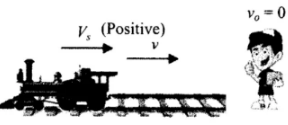Apparent frequency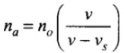It is clear that na > n0:
When the train is going away from the observer.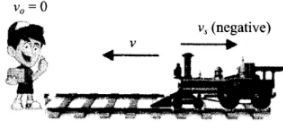Apparent frequency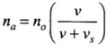It is clear that na > no
Hence, the expected curve is (c).

MULTIPLE CHOICE QUESTIONS II

Q.11. A transverse harmonic wave on a string is described by y(x, t) = 3.0 sin (36t + 0.018x + π/4)
where x and y are in cm and t is in sec. The positive direction of x is from left to right.
(a) The wave is travelling from right to left.
(b) The speed of the wave is 20 m/s.
(c) Frequency of the wave is 5.7 Hz.
(d) The least distance between two successive crests in the wave is 2.5 cm.
Ans.
(a,b,c)
Solution.
The general equation of a plane progressive wave with initial phase is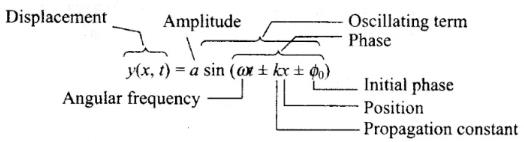Various forms of progressive wave function: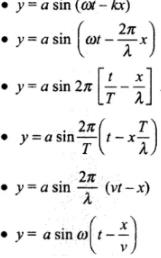Given equation is
y(x, t) = 3.0 sin (36 t + 0.018x + π/4)
Option (a): Since there is +ve sign between ωt and kx, the wave travels from right to left ( the positive direction of x is from left to right). Hence it is correct.
Option (b): Speed of the wave,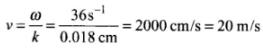Hence it is correct.
Option (c): Frequency of the wave,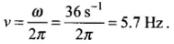Hence it is correct.
Option (d): Least distance between two successive crests,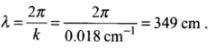Hence it is wrong.

Q.12. The displacement of a string is given by y(x, t) = 0.06 sin(2πx/3) cos(120πt) where x and y are in m and t in sec. The length of the string is 1.5 m and its mass is 3.0 x 10-2 kg.
(a) It represents a progressive wave of frequency 60 Hz.
(b) It represents a stationary wave of frequency 60 Hz.
(c) It is the result of superposition of two waves of  wavelength 3 m, frequency 60 Hz each travelling with a speed of 180 m/s in opposite direction.
(d) Amplitude of this wave is constant.
Ans.
(b, c)
Solution.
Standing Waves or Stationary Waves:
When two sets of progressive wave trains of same type (both longitudinal or both transverse) having the same amplitude and same time period/ frequency/wavelength travelling with same speed along the same straight line in opposite directions superimpose, a new set of waves are formed. These are called stationary waves or standing waves.
Suppose that the two superimposing waves are incident wave y1 = a sin(ωt - kx) and reflected wave y2 = a sin(ωt + kx).
(As y2 is the displacement due to a reflected wave from a free boundary).
Then by principle of superposition
y = y1 + y2 = a[sin(ωt - kx) + sin (ωt + kx)]
which gives the standard equation of stationary wave.
y(x, t) = 2a sin (kx) cos(ωt)
Given equation is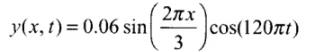Option (a): Comparing with a standard equation of stationary wave y(x, t) = 2a sin (kx) cos(ωt)
Clearly, the given equation belongs to stationary wave. Hence, option (a) is not correct.
Option (b): By comparing, ω = 120π
⇒ 2π= 120π
⇒ f = 60 Hz.
Hence it is correct.
Option (c):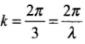⇒ λ = wavelength = 3 m
Frequency = f = 60 Hz
Speed = v = fλ = (60 Hz)(3 m) = 180 m/s. Hence it is correct.
Option(d): Since in stationary wave, all particles of the medium execute SHM with varying amplitude nodes. Hence, option (d) is not correct.

Q.13. Speed of sound waves in a fluid depends upon
(a) Directly on density of the medium.
(b) Square of Bulk modulus of the medium.
(c) Inversely on the square root of density.
(d) Directly on the square root of bulk modulus of the medium
Ans.
(c, d)
Solution.
We define the speed of sound waves in a fluid as,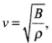Here B is the Bulk modulus and ρ  is density of the medium.
It means, v ∝ 1/√ρ
[ ∴ for any fluid, B = constant]
and v ∝√B
[ ∵ for medium ρ = constant]
Hence, options (c) and (d) are correct.

Q.14. During propagation of a plane progressive mechanical wave
(a) All the particles are vibrating in the same phase.
(b) Amplitude of all the particles is equal.
(c) Particles of the medium executes S.H.M.
(d) Wave velocity depends upon the nature of the medium
Ans.
(b, c, d)
Solution.
Characteristics of wave motion:

• When a wave motion passes through a medium, particles of the medium only vibrate simple harmonically about their mean position. They do not move with the disturbance.
• Medium particles oscillate with same frequency and also the amplitude of oscillation of all the particles is equal. All the particles marked as 1, 2, 3,4 and 5 oscillate with the same frequency.
• There is a continuous phase difference amongst successive particles of the medium, i.e. particle 2 starts vibrating slightly later than particle 1 and so on.
• The velocity of the particle during their vibration is different at different positions.
• The velocity of wave motion through a particular medium is constant. It depends only on the nature of medium not on the frequency, wavelength or intensity.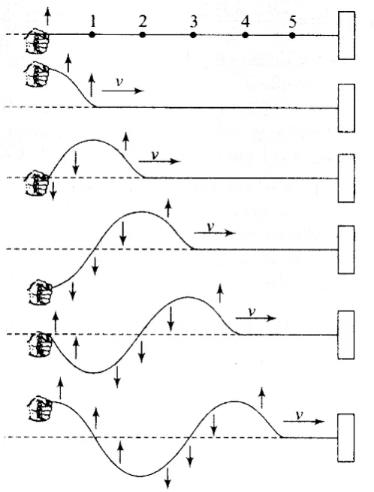Option (a): Clearly, the particles 1, 2 and 3 are having different phase.
Option (b) and (c): Particles of the wave shown in the figure executes SHM with same amplitude.
Option (d): The wave velocity of mechanical wave depends only on elastic and inertia property of medium for a progressive wave propagating in a fluid. Hence wave velocity depends upon the nature of the medium.
Speed = v =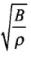Hence,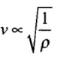[ ∵ B is constant]
As ρ depends upon nature of the medium, hence v also depends upon the nature of the medium.

Q.15. The transverse displacement of a string (clamped at its both ends) is given by y (x,t) = 0.06 sin (2πx/3) cos (120πt).
All the points on the string between two consecutive nodes vibrate with
(a) Same frequency
(b) Same phase
(c) Same energy
(d) Different amplitude
Ans.
(a, b, d)
Solution.

• The points for which amplitude is minimum are called nodes in a stationary wave, nodes are equally spaced at a distance λ/2.
• The points for which amplitude is maximum are called antinodes. Like nodes, antinodes are also equally spaced with spacing (λ/2) Furthermore, nodes and antinodes are alternate with spacing (λ/4).
• The nodes divide the medium into segments (or loops). All the particles in a segment vibrate in same phase, but in opposite phase with the particles in the adjacent segment. Twice in one period all the particles pass through their mean position simultaneously with maximum velocity (A at), the direction of motion being reversed after each half cycle.
Given equation is
y(x, t) = 0.06 sin(2π/3 (x)) cos(120πt)
This represents stationary wave. All the points on the string between two consecutive nodes vibrate with same frequency, But are having different amplitudes of 0.06 sin(2π/3 (x)) and because of different amplitudes they are having different energies.
Also all the particles between two nodes they are having same phase of (120πt) at a given time.

Q.16. A train, standing in a station yard, blows a whistle of frequency 400 Hz in still air. The wind starts blowing in the direction from the yard to the station with a speed of 10 m/s. Given that the speed of sound in still air is 340 m/s,
(a) The frequency of sound as heard by an observer standing on the platform is 400 Hz.
(b) The speed of sound for the observer standing on the platform is 350 m/s.
(c) The frequency of sound as heard by the observer standing on the platform will increase.
(d) The frequency of sound as heard by the observer standing on the platform will decrease.
Ans.
(a, b)
Solution.
When the wind is blowing in the same direction as that of sound wave, then net speed of the wave is sum of speed of sound wave and speed of the wind.
Given, f0 = 400 Hz, v = 340 m/s Speed of wind vw = 10 m/s
Option (a): As there is no relative motion between the source and observer, hence frequency observed will be the same as natural frequency f0 = 400 Hz
Option (b): When the wind is blowing in the same direction as that of sound wave, then net speed of the wave is sum of speed of sound wave and speed of the wind. The speed of sound v = v + vw
= 340+ 10 = 350 m/s
Option (b) and (c): There will be no change in frequency because there is no relative motion between source and observer. Hence (c) and (d) are incorrect.

Q.17. Which of the following statements are true for a stationary wave?
(a) Every particle has a fixed amplitude which is different from the amplitude of its nearest particle.
(b) All the particles cross their mean position at the same time.
(c) All the particles are oscillating with same amplitude.
(d) There is no net transfer of energy across any plane.
(e) There are some particles which are always at rest.
Ans.
(a, b, d, e)
Solution.
Consider the equation of a stationary wave
y(x, t) = 2a sin (kx) cos (wt)
Option (a): In stationary wave any particle at a given position have amplitude 2a sin kx.
Option (b): The time period of oscillation of all the particles is same, hence all the particles cross their mean position at the same time.
Option (c): Amplitude of all the particles are 2a sin kx which is different for different particles at different values of x.
Options (d) and (e): Nodes are the points which is always at rest hence no transfer of energy across the nodes. It means the energy is a stationary wave is confined between two nodes.

Q.1. A sonometer wire is vibrating in resonance with a tuning fork. Keeping the tension applied same, the length of the wire is doubled. Under what conditions would the tuning fork still be is resonance with the wire?
Ans.
Wire of sonometer is twice the length which it vibrates in its second harmonic. Thus, if the tuning fork resonates at L, it will resonate at 2L. This can be explained as below:
The frequency of sonometer is given by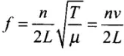(n = number of loops)
For a given sonometer velocity of wave will be constant. If after changing the length of wire the tuning fork still be in resonance with the wire. Then, n/L = constant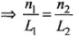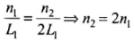Hence, when the wire is doubled the number of loops also get doubled to produce the resonance. That is it resonates in second harmonic.

Q.2. An organ pipe of length L open at both ends is found to vibrate in its first harmonic when sounded with a tuning fork of 480 Hz. What should be the length of a pipe closed at one end, so that it also vibrates in its first harmonic with the same tuning fork?
Ans.
As, the medium and frequency and number of harmonic in open and closed pipes are same, so number of nodes and (wave), in both cases will be same.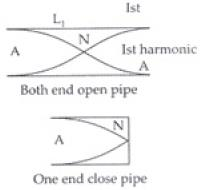In both end open pipe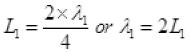and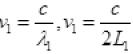In one end open pipe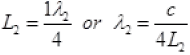As medium and turning fork in both cases are same v1 = vand c1 = c2 = c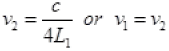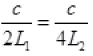or 4L2 = 2L1 or L2 = L1/2
So the length of one end closed pipe will be half of both end open pipe for resonant Ist harmonic with same frequency.

Q.3. A tuning fork A, marked 512 Hz, produces 5 beats per second, where sounded with another unmarked tuning fork B. If B is loaded with wax the number of beats is again 5 per second. What is the frequency of the tuning fork B when not loaded?
Ans.
vA = 512, ∵ v0 = vA ∼ vB no. of frequency of beats
So in first case v1 = 5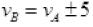When B is loads frequency of B is v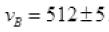i.e., vB either 507 or 517.
On load in frequency of B decreased 507 to lower value of number of beats will increase so vB ≠ 507. Now if vB = 517 then its frequency decrease by 10 Hz then number of beats will also be same as 512 – 507 = 5. So frequency of tuning fork when unloaded is 517.

Q.4. The displacement of an elastic wave is given by the function y = 3 sin ωt + 4 cos ωt.  where y is in cm and t is in second. Calculate the resultant amplitude.
Ans.
Given, displacement of the wave
y = 3 sin ωx + 4 cos ωx
Let us assume, 3 = A cos θ   ...(i)
3 = Acos θ        …(ii)
On dividing Eq. (ii) by Eq. (i)
tan θ = 4/3 => ϕ= tan-1(4/3)
Squaring and adding equations (i) and (ii),
A2 cos2 θ + A2 sin2 θ = 32 + 42
A2 (cos2 θ + sin2 θ) = 25
A2 = 25 ⇒ A = 5. Hence, amplitude = 5 cm
Frequency of vibrations,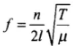Mass per unit length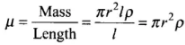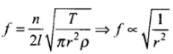or f ∝ 1/r
Hence, when radius is tripled, the frequency will be 1/3 rd of previous value.

Q.5. A sitar wire is replaced by another wire of same length and material but of three times the earlier radius. If the tension in the wire remains the same, by what factor will the frequency change?
Ans.
The wire is stretched both end so frequency of stretched wire is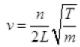As number of harmonic n, length L and tension (T) are kept same in both cases.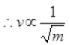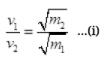Mass per unit length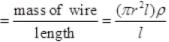m = πr2ρ
As material of wire is same.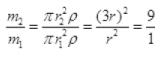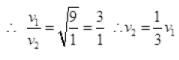So the frequency of sitar reduced by 1/2 of previous value.

Q.6. At what temperatures (in oC) will the speed of sound in air be 3 times its value at 0oC?
Ans.
We know that speed of sound in air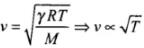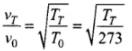But it is given,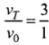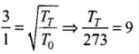∴ TT = 273 x 9 = 2457 K
= 2457 - 273 = 2184ºC

Q.7. When two waves of almost equal frequencies n1 and n2 reach at a point simultaneously, what is the time interval between successive maxima?
Ans.
If two waves of almost equal frequencies interfere, they are producing beats.
Let n1 > n2
Beat frequency fbeat = n1 - n2
∴ Time period of beats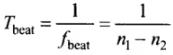This time period will be equal to the time interval between successive maxima.

Offer running on EduRev: Apply code STAYHOME200 to get INR 200 off on our premium plan EduRev Infinity!

,

,

,

,

,

,

,

,

,

,

,

,

,

,

,

,

,

,

,

,

,

;